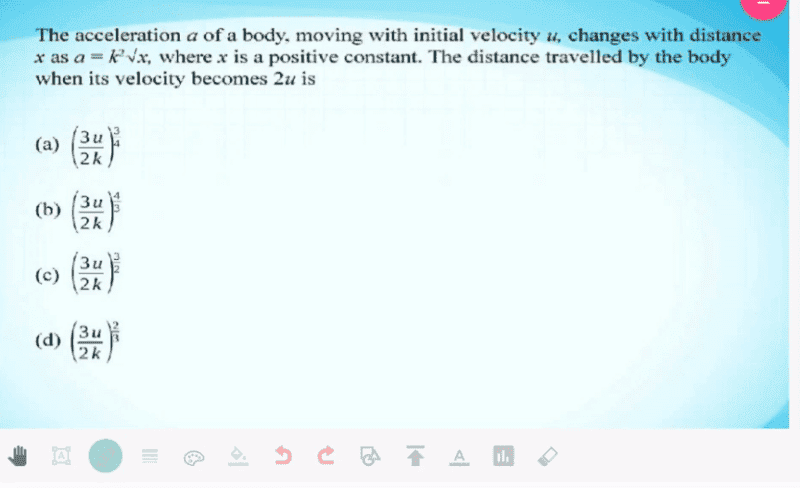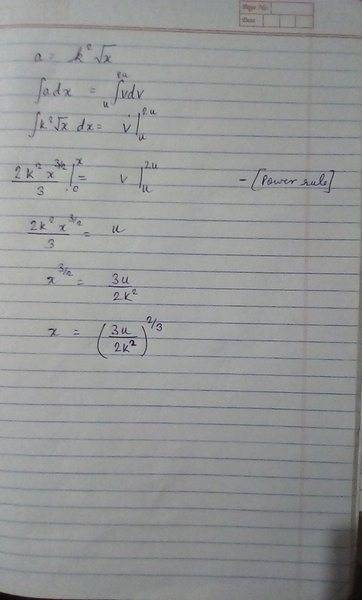# Having a problem in steps while solving integrals

prakhargupta3301

## Homework Statement

The problem is attached. I'm new to these problems (calculus).
I'm not getting my answer as any of the options. I need your help to know whether me or the slide is wrong.x_x[/B]

## The Attempt at a Solution#### Attachments

Homework Helper
Gold Member
What is ∫v.dv?

•prakhargupta3301
Dr Dr news
The slide is wrong in that x is not constant, k is constant. Your formulation is correct up to ∫ v dv as pointed out by haruspex.

•prakhargupta3301
prakhargupta3301
What is ∫v.dv?
It is an equation to solve kinematic equations.
a=dv/dt 1
v=dx/dt 2
Divide 1 by 2
a/v= dv/dt⋅dt/dx
a/v=dv/dx
a⋅dx=v⋅dv
Integrate LHS and RHS and you get 3rd equation of motion:
0x∫a⋅dx=uv∫v⋅dv
a⋅x0x=v2|uv
ax-0=v2/2-u2/2
2ax=v2-u2

prakhargupta3301
The slide is wrong in that x is not constant, k is constant. Your formulation is correct up to ∫ v dv as pointed out by haruspex.
So, am I correct or not ? This was quite an ambiguous reply..
If not, can you point out the mistake clearly and correction. Please?

Dr Dr news
Your LHS (2/3) k^2 x^(3/2) is OK the RHS as you have corrected is v^2 / 2 with an upper limit of 2u and a lower limit of u.

•prakhargupta3301
•